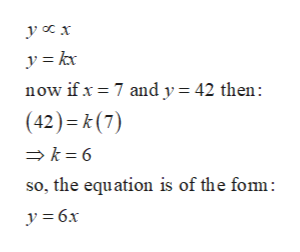# If y is directly proportional to x, and y=42 when x=7, find the constant of proportionality and write a formula for y in terms of x.  y= Use the formula to find x when y is 24x=

Question
3 views
If y is directly proportional to x, and y=42 when x=7, find the constant of proportionality and write a formula for y in terms of x.

y=

Use the formula to find x when y is 24
x=
check_circle

Step 1

Given that y is directly proportional to x or y x.

So we Can write this in other form as:help_outlineImage Transcriptionclosey a x now if x 7 and y= 42 then (42) k(7) k 6 so, the equation is of the fom y = 6x fullscreen
Step 2

So, the constant is 6 and the formula for y in term of x is y = 6x

Step 3

Use the formula to find x when y is 24:

Consider y = 24 then we have:

...

### Want to see the full answer?

See Solution

#### Want to see this answer and more?

Solutions are written by subject experts who are available 24/7. Questions are typically answered within 1 hour.*

See Solution
*Response times may vary by subject and question.
Tagged in

### Functions